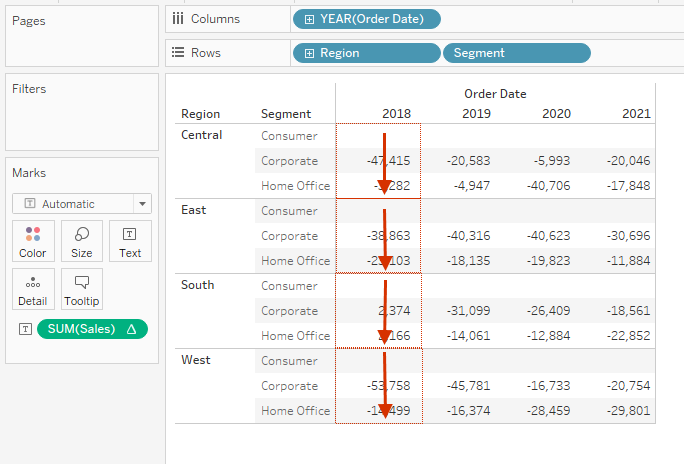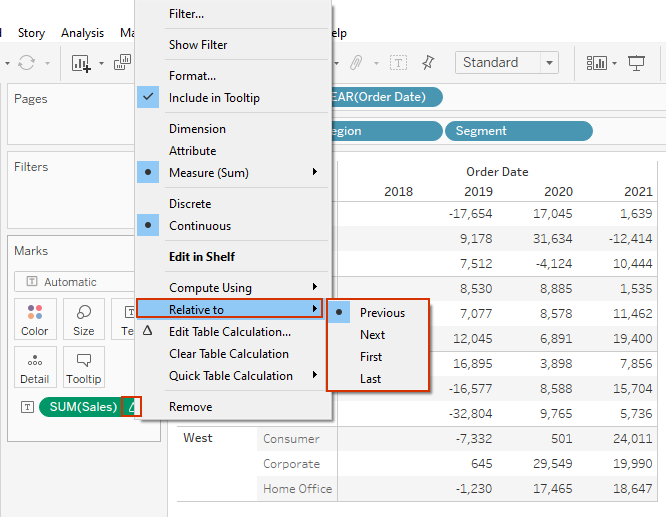Search
• Bernard

# Compute Using - for Tableau table calculations.Table calculations are special types of calculated fields that computes on values in a visualization. They are calculated based on what is in the current visualization and do not consider measures or dimensions that are filtered out of the visualization.

Table calculation can be used in.

• Transforming values into rankings.

• Transforming values to show running totals.

• Transforming values to show percent of total.

• Transforming values to show percent difference.

• Transforming values to show moving average etc.

When you add a table calculation, you must use all the dimensions in the level of detail either for partitioning (defining the scope of data the calculation is performed on) or for addressing (determining the direction of the calculation).

Partitioning fields break the view into multiple sub-views and then the table calculation is applied to the marks within each partition – with the direction in which the calculation moves being determined by the addressing fields.

### Let’s get practical by creating a view.

In this case, using Sample-superstore data source.

• Drag Order Date to columns.

• Drag Region and Segment to rows.

• Add Sales to the label shelf.Next add a table calculation ‘Difference’ as shown below.### Table (across)

Executing this (by default) computes, the difference across the column YEAR(Order Date) for every row (Segment).With ‘Compute Using’ option, we can customize the direction in which our table calculations are computed.The views below, will help you understand how these different option under ‘Compute Using’ are executed.

### Table (down)

The calculation is computed down the rows Segment for every column YEAR(Order Date).### Table (across then down)

The calculation is computed across the columns YEAR(Order Date), then down a row Segment, then across the columns again for the entire table.### Table (down then across)

This calculation is computed down the rows segment, then across a column YEAR(Order Date), and then down rows again.### Pane (down)

This calculation is computed down rows Segment for a single pane.### Pane (across then down)

This calculation is computed across the columns YEAR(Order Date) for the length of pane, then down a row Segment and then across columns for the length of the pane.### Pane (down then across)

This calculation is computed down the rows Segment for the length of the pane, then across a column YEAR(Order Date) and then down the length of the pane again.### Cell

This calculation is computed within a single cell.### Compute using specific dimensions.

This calculation is computed within the dimension you specify.

In the following view, the calculation has been computed using dimension ‘Region’### Relative to

Another option you should note when working with table calculations is ‘Relative to’ which defines the point of reference when computing your table calculation. Some of the options you can use include, previous, next, first, last.I hope this post was helpful to you. To receive more of the Tableau tips kindly join our mailing list by subscribing below.